# Penrose transform

A construction from complex integral geometry, its definition very much resembling that of the Radon transform in real integral geometry. It was introduced by R. Penrose in the context of twistor theory [a4] but many mathematicians have introduced transforms which may now be viewed in the same framework.

In its most general formulation, one starts with a correspondence between two spaces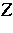and. In many caseswill be a complex manifold but it can also be a CR-manifold or, indeed, a manifold with an involutive or formally integrable structure so that it makes sense to say that a smooth submanifold ofis complex. The important aspect of the correspondence is that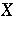parameterizes certain compact complex submanifolds of(called cycles).

In the classical case,andwith the obvious incidence relation. Thus, a point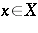gives a complex projective linein. This correspondence also has a real form, namely the fibration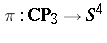. It can be defined by regarding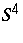as quaternionic projective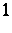-space with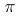taking a complex line to its quaternionic span, having chosen an identification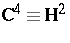. See [a3] for the Penrose transform in this real setting. There are several examples from representation theory, the prototype being due to W. Schmid [a5] with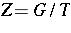and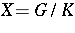. Here,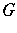is a semi-simple Lie group with maximal torus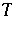and maximal compact subgroup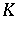assumed to have the same rank as(cf. Lie group, semi-simple). In complex geometry there is also the Andreotti–Norguet transform [a1], with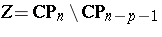and with as cycles the linearly embedded subspaces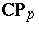.

In all of these transforms, one starts with a cohomology classon. Ifis complex, then it is a Dolbeault class (cf. Differential form), ifis CR, then it is a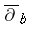-cohomology class (cf. de Rham cohomology), and so on. Often, the coefficients are twisted by a holomorphic or CR-bundle on. In its simplest form, the Penrose transform simply restricts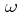to the cycles. Since each cycle is a compact complex manifold, the restricted cohomology is a finite-dimensional vector space. Supposing that the dimension of this vector space is constant as one varies the cycle, this gives a vector bundle onand the restriction ofto each cycle gives a section of this bundle.

In the classical case, or its real form, suppose that one considers a Dolbeault cohomology classof degree one with coefficients in, where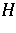is the hyperplane bundle on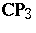. Since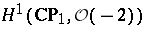is one-dimensional, the resulting bundle onoris a line bundle. The Penrose transform ofis the section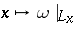in the complex case, or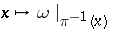in the real case. For the Andreotti–Norguet transform one takes as coefficients, the bundle of holomorphic one-forms. Then, in view of the canonical isomorphism, the transform gives rise to a function.

In any case, there are preferred local trivializations of the bundles involved and one can ask whether the resulting function is general. It will be holomorphic in the complex case and smooth in the real case, but there are further conditions. Unlike the analogous Radon transform, these conditions apply locally, so that, for example, there results an isomorphism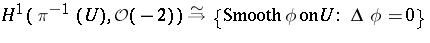for any open subset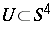, where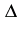is the conformally invariant Laplacian on(cf. Laplace operator). Similarly, the Penrose transform interprets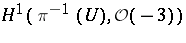as solutions of the Dirac equation on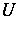. The range of the Andreotti–Norguet transform consists of functions in the kernel of the Paneitz operator, a conformally invariant fourth-order operator. The range of Schmid's transform, when the coefficients are in a line bundle induced from an anti-dominant integral weight sufficiently far from the walls and cohomology is taken in degree equal to the complex dimension of the cycles, is the kernel of the Schmid operator.

In several cases, these transforms have appeared much earlier as integral transforms of holomorphic functions. For example, on this level, the Penrose description of harmonic functions is due to H. Bateman in 1904. A proper understanding, however, arises only when these holomorphic functions are viewed as Čech cocycles representing a cohomology class. See [a2] for further discussion.

There is a "non-linear" version of the Penrose transform, due to R.S. Ward [a6] and known as the Ward transform. In the classical case, it identifies certain holomorphic vector bundles onwith solutions of the self-dual Yang–Mills equations (cf. Yang–Mills field) on.

How to Cite This Entry:
Penrose transform. M.G. Eastwood (originator), Encyclopedia of Mathematics. URL: http://encyclopediaofmath.org/index.php?title=Penrose_transform&oldid=12831
This text originally appeared in Encyclopedia of Mathematics - ISBN 1402006098